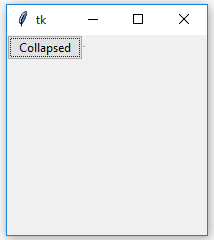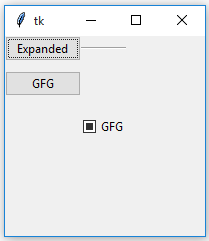Related Articles
Collapsible Pane in Tkinter | Python
• Last Updated : 18 Oct, 2019

A collapsible pane, as the name suggests, is a pane which can be collapsed. User can expand pane so that they can perform some task and when task is completed, pane can be collapsed.
In Tkinter, Collapsible pane is a container with an embedded button-like control which is used to expand or collapse this container.

Prerequisites:

```Frame Class
Checkbutton Class
Styling in widgets
configure() method
```

`CollapsiblePane `class –
CollapsiblePane widget is used to store any other widgets inside of it. It can be toggled on or off, so widgets inside of it aren’t always shown.

Parameters:
`parent =` The parent of the widget.
`expanded_text =` The text shown on the Button when the pane is open.
`collapsed_text =` The text shown on the Button when the pane is closed.

Functions:
`_activate() =` Checks the value of variable and shows or hides the Frame.
`toggle() = `Switches the LabelFrame to the opposite state.

Widgets:
`self_button =` The Button that toggles the Frame.
`frame =` The Frame that holds the widget.
`_separator =` The Separator.

 `# Implementation of Collapsible Pane container`` ` `# importing tkinter and ttk modules``import` `tkinter as tk``from` `tkinter ``import` `ttk`` ` `class` `CollapsiblePane(ttk.Frame):``    ``"""``     ``-----USAGE-----``    ``collapsiblePane = CollapsiblePane(parent, ``                          ``expanded_text =[string],``                          ``collapsed_text =[string])`` ` `    ``collapsiblePane.pack()``    ``button = Button(collapsiblePane.frame).pack()``    ``"""`` ` `    ``def` `__init__(``self``, parent, expanded_text ``=``"Collapse <<"``,``                               ``collapsed_text ``=``"Expand >>"``):`` ` `        ``ttk.Frame.__init__(``self``, parent)`` ` `        ``# These are the class variable``        ``# see a underscore in expanded_text and _collapsed_text``        ``# this means these are private to class``        ``self``.parent ``=` `parent``        ``self``._expanded_text ``=` `expanded_text``        ``self``._collapsed_text ``=` `collapsed_text`` ` `        ``# Here weight implies that it can grow it's``        ``# size if extra space is available``        ``# default weight is 0``        ``self``.columnconfigure(``1``, weight ``=` `1``)`` ` `        ``# Tkinter variable storing integer value``        ``self``._variable ``=` `tk.IntVar()`` ` `        ``# Checkbutton is created but will behave as Button``        ``# cause in style, Button is passed``        ``# main reason to do this is Button do not support``        ``# variable option but checkbutton do``        ``self``._button ``=` `ttk.Checkbutton(``self``, variable ``=` `self``._variable,``                            ``command ``=` `self``._activate, style ``=``"TButton"``)``        ``self``._button.grid(row ``=` `0``, column ``=` `0``)`` ` `        ``# This wil create a seperator``        ``# A separator is a line, we can also set thickness``        ``self``._separator ``=` `ttk.Separator(``self``, orient ``=``"horizontal"``)``        ``self``._separator.grid(row ``=` `0``, column ``=` `1``, sticky ``=``"we"``)`` ` `        ``self``.frame ``=` `ttk.Frame(``self``)`` ` `        ``# This will call activate function of class``        ``self``._activate()`` ` `    ``def` `_activate(``self``):``        ``if` `not` `self``._variable.get():`` ` `            ``# As soon as button is pressed it removes this widget``            ``# but is not destroyed means can be displayed again``            ``self``.frame.grid_forget()`` ` `            ``# This will change the text of the checkbutton``            ``self``._button.configure(text ``=` `self``._collapsed_text)`` ` `        ``elif` `self``._variable.get():``            ``# increasing the frame area so new widgets``            ``# could reside in this container``            ``self``.frame.grid(row ``=` `1``, column ``=` `0``, columnspan ``=` `2``)``            ``self``._button.configure(text ``=` `self``._expanded_text)`` ` `    ``def` `toggle(``self``):``        ``"""Switches the label frame to the opposite state."""``        ``self``._variable.``set``(``not` `self``._variable.get())``        ``self``._activate()`

Program to demonstrate use of CollapsiblePane –

 `# Importing tkinter and ttk modules``from` `tkinter ``import` `*` `from` `tkinter.ttk ``import` `*`` ` `# Importing Collapsible Pane class that we have``# created in separate file``from` `collapsiblepane ``import` `CollapsiblePane as cp`` ` `# Making root window or parent window``root ``=` `Tk()``root.geometry(``'200x200'``)`` ` `# Creating Object of Collapsible Pane Container``# If we do not pass these strings in``# parameter the the defalt strings will appear``# on button that were, expand >>, collapse <<``cpane ``=` `cp(root, ``'Expanded'``, ``'Collapsed'``)``cpane.grid(row ``=` `0``, column ``=` `0``)`` ` `# Button and checkbutton, these will``# appear in collapsible pane container``b1 ``=` `Button(cpane.frame, text ``=``"GFG"``).grid(``            ``row ``=` `1``, column ``=` `2``, pady ``=` `10``)`` ` `cb1 ``=` `Checkbutton(cpane.frame, text ``=``"GFG"``).grid(``                  ``row ``=` `2``, column ``=` `3``, pady ``=` `10``)`` ` `mainloop()`

Output:Attention geek! Strengthen your foundations with the Python Programming Foundation Course and learn the basics.

To begin with, your interview preparations Enhance your Data Structures concepts with the Python DS Course. And to begin with your Machine Learning Journey, join the Machine Learning – Basic Level Course

My Personal Notes arrow_drop_up